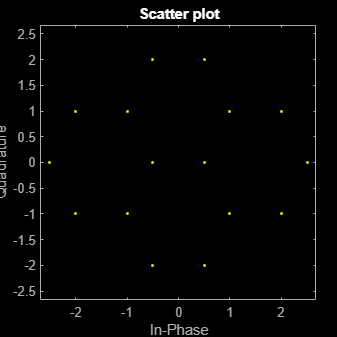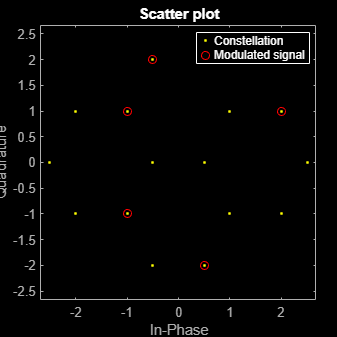Documentation

This is machine translation

Mouseover text to see original. Click the button below to return to the English version of the page.

genqamdemod

Syntax

```z = genqamdemod(y,const) ```

Description

`z = genqamdemod(y,const)` demodulates the complex envelope `y` of a quadrature amplitude modulated signal. The complex vector `const` specifies the signal mapping. If `y` is a matrix with multiple rows, the function processes the columns independently.

Examples

collapse all

Create the points that describe a hexagonal constellation.

```inphase = [1/2 1 1 1/2 1/2 2 2 5/2]; quadr = [0 1 -1 2 -2 1 -1 0]; inphase = [inphase;-inphase]; inphase = inphase(:); quadr = [quadr;quadr]; quadr = quadr(:); const = inphase + 1i*quadr;```

Plot the constellation.

`h = scatterplot(const);`Generate input data symbols. Modulate the symbols using this constellation.

```x = [3 8 5 10 7]; y = genqammod(x,const);```

Demodulate the modulated signal, `y`.

`z = genqamdemod(y,const);`

Plot the modulated signal in same figure.

```hold on; scatterplot(y,1,0,'ro',h); legend('Constellation','Modulated signal');```Determine the number of symbol errors between the demodulated data to the original sequence.

`numErrs = symerr(x,z)`
```numErrs = 0 ```